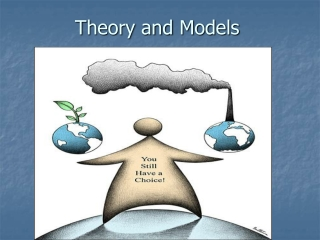# Theory and Models - PowerPoint PPT PresentationDownload PresentationTheory and Models

Theory and Models
Download Presentation## Theory and Models

- - - - - - - - - - - - - - - - - - - - - - - - - - - E N D - - - - - - - - - - - - - - - - - - - - - - - - - - -
##### Presentation Transcript

1. Theory and Models

2. Why Understand Economics? Automakers, and the Strong Dollar of 1986 1986: \$1 ===> 240 yen 1988: \$1 ===> 120 yen 1994: \$1 ===> 100 yen 1995: \$1 ===> 100 yen 1996: \$1 ===> 116 yen 1997: \$1 ===> 134 yen

3. Why Understand Economics? Big Three U.S. Automakers, and the Strong Dollar of 1986 1986: \$1 ===> 240 yen 1988: \$1 ===> 120 yen 1994: \$1 ===> 100 yen 1995: \$1 ===> 100 yen 1996: \$1 ===> 116 yen 1997: \$1 ===> 134 yen

4. Why Understand Economics? One Third of the Iran Population was Born Between 1361 to 1969 “Baby Boomers” 1395: 36 to 54 years old How will this aging of our population affect our economy in the future?

5. Theory and Models Economics is a social science that makes use of similar methodology used by the “hard” sciences (biology, physics, etc.) The “hard” sciences refer to this method as the “scientific method”, in economics we will refer to this method as the “economic method”

6. The Scientific Method Step 1: The observation of phenomena, Step 2: The formulation of a hypothesis concerning the phenomena, Step 3: Experimentation to demonstrate the truth or falseness of the hypothesis, Step 4: Develop a conclusion that validates or modifies the hypothesis.

7. The Economic Method1 Step 1: Identify and state the problem Step 2: Apply the relevant economic model Step 3: Identify the solutions Step 4: Evaluate the solutions Step 5: Select and implement a solution 1Adaped from Mabry and Ulbrich, “Introduction to Economic Principles, 1989.

8. Theory and Models Models: Simplified representations of the “real world” that we use to help us understand, explain, and predict economic events in the real world.

9. Theory and Models • No model captures every little detail and inter-relationship that exists • Models are abstractions from reality

10. Theory and Models A Good Model should capture only the essential relationships that are sufficient to analyze a particular problem, or answer a particular question. A Model is the guideline we follow to analyze economic problems and predict outcomes.

11. Theory and Models Questions: How do consumers respond when the price of a commodity changes? What choices do they have? What choices do they make?

12. Theory and Models Dove season opens soon, and we want some shotgun shells. How we will respond to a price of 10000 Toman per box of 25 shells? There are probably thousands of determinants affecting how each consumer will respond to this high price.

13. Theory and Models Many of these determinants were left out of the model we used to answer the questions above. Our class model probably included: • The price of the specific commodity • The income of the consumer • The price of substitutes for the commodity

14. Theory and Models These 3 determinants do a good job of explaining the behavior of consumers with respect to a price change. Later we will learn that there are in excess of 10 determinants that affect consumer demand.

15. Theory and Models An economic model is nothing more than a set of definitions, assumptions, and hypotheses that are put together in a manner that expresses the relationships of certain observed events in a meaningful way.

16. The Consumption-Production Model In Equilibrium: PRICE CONSUMPTION = PRODUCTION INVENTORIES

17. In Disequilibrium: P C < Pr Iv How should a manager respond in this case?

18. In Disequilibrium: • What if production process is at full capacity? • What if production process is at less than full capacity?

19. In Disequilibrium: P C > Pr Iv How should a manager respond in this case?

20. In Disequilibrium: • What if production process is at full capacity? • What if production process is at less than full capacity?

21. Short Run vs. Long Run? • Short Run1: a period of time that is not long enough to allow change to certain economic conditions that a decision maker may face. • Long Run1: a period of time long enough for all important information and choices to be available to a decision maker 1Mabry and Ulbrich, “Introduction to Economic Principles, 1989

22. References: • N.c.State university-College of Agriculture and Life science –Dr. herman_sampson